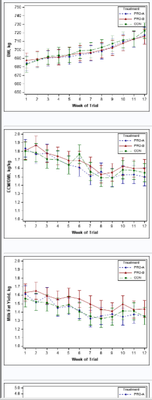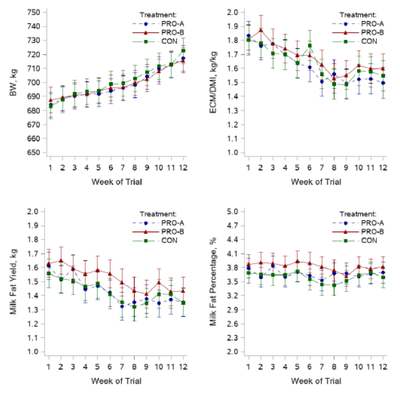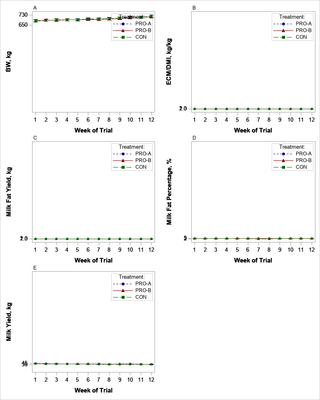## PROC template GraphI have attached the data if it would be useful in your helping me achieve this task.

``````Data Selected;
Set mylibrary.Mean_Comparison_Proc;
Period2 = input(Period, 2.);
Drop Period;
rename Period2=Period;
run;
Proc Sort Data=Selected;
by resp_name Treatment Period;
run;``````

I created the above individual graphs using SGPLOT within a macro, with the below code:

``````Proc Sql noprint;
select count(distinct resp_name) into :obs from Selected;
select distinct resp_name into :resp1-:resp5 from Selected;
Quit;

%Macro TestSee;
%do i = 1 %to &obs;
%Let value1=(650 to 750 by 10); %Let value2=(1.0 to 2.0 by 0.1); %Let value3=(1 to 2.0 by 0.1); %Let value4=(2 to 5 by 0.1); %Let value5=(35 to 45 by 1);
%Let ylabel1=BW, kg; %Let ylabel2=ECM/DMI, kg/kg; %Let ylabel3=Milk Fat Yield, kg; %Let ylabel4=Milk Fat Percentage, %; %Let ylabel5=Milk Yield, kg;
%Let xlabel=Week of Trial;
proc format;
value \$treatmentFmt
"Bov" = "PRO-A"
"BovC" = "PRO-B"
"Control" = "CON"
;
ods html style=listing; *In SAS 9.4 you need a style like LISTING to see different symbols;
Proc SGPLOT data = Selected noautolegend;
format treatment \$treatmentFmt.;
where resp_name="&&resp&i";
styleattrs datasymbols=(CircleFilled triangleFilled SquareFilled) datalinepatterns=(ShortDash Solid MediumDash) datacontrastcolors=(darkblue darkred darkgreen);
Series x=Period y=estimate/ group=Treatment grouplc=Treatment groupmc=Treatment markers lineattrs=(thickness=1) name="Treatment";
Scatter x=Period y=estimate/ group=Treatment name="a" YErrorUpper=Upper YErrorLower=Lower;*groupdisplay=cluster clusterwidth=0.6 datalabel=MSgroup datalabelattrs=(size=9pt color=gray);
yaxis label="&&ylabel&i" Labelattrs=(Family=Arial Size=11 weight=Bold ) valueattrs=(size=10) values=&&value&i;
xaxis discreteorder=data label="&xlabel." Labelattrs=(Family=Arial Size=11 weight=Bold ) valueattrs=(size=10) values=(1 to 12 by 1);
keylegend "Treatment"/ title="Treatment:" location=inside position=topright across=1;
run;
%end;
Ods html CLOSE;
%mend;
%TestSee;``````

What i however need is a grid of all 5 graphs with independent axis, all labelled as i did with the above graph. I did research on Proc template and it seems it could really do the job for me, but i still couldn't figure out how to make this work. I will really appreciate your help guys. Thank you.

1 ACCEPTED SOLUTION

Accepted Solutions

## Re: PROC template Graph

Ah yes, I forgot to put the VIEWMIN and VIEWMAX values in for the y-axis. I've added several macro variables for min/max values to use in the VIEWMIN/VIEWMAX options below.  There are more streamlined ways to do this but I'm making it more verbose to read easier to what I'm doing.  Look for the VIEWMIN and VIEWMAX options below as this sets what is visible in the axis.

As for the ylabel being spaced a bit.  This is because it doesn't exist in the same space as the y-axis tick marks.  The wider the tickmarks are the further the y-axis label will be.  It won't look as bad when there are actual tick values showing up appropriately, but the only real way to make it closer is to use annotation which is another animal.  I can explain how to do that if needed, but try the changes below to see if that helps at all.

Here is some updated code:

``````
%Macro TestSee;
proc format;
value \$treatmentFmt
"Bov" = "PRO-A"
"BovC" = "PRO-B"
"Control" = "CON"
;
run;

PROC TEMPLATE;
DEFINE STATGRAPH _grid;
BEGINGRAPH / designheight=10in designwidth=8in;

%Let value1=start=650 end=750 increment=10; %let min1=650; %let max1=750;%Let value2=start=1.0 end=2.0 increment=0.1; %let min2=1; %let max2=2;%Let value3=start=1 end=2.0 increment=0.1; %let min3=1; %let max3=2;%Let value4=start=2 end=5 increment=0.1; %let min4=2; %let max4=5;%Let value5=start=35 end=45 increment=1; %let min5=35; %let max5=45;
%Let ylabel1=BW, kg; %Let ylabel2=ECM/DMI, kg/kg; %Let ylabel3=Milk Fat Yield, kg; %Let ylabel4=Milk Fat Percentage, %; %Let ylabel5=Milk Yield, kg;
%Let xlabel=Week of Trial;

DISCRETEATTRMAP name='styles' / ignorecase=true;
value 'PRO-A' / markerattrs=(symbol=circlefilled color=darkblue) lineattrs=(pattern=shortdash color=darkblue);
value 'PRO-B' / markerattrs=(symbol=trianglefilled color=darkred) lineattrs=(pattern=solid color=darkred);
value 'CON' / markerattrs=(symbol=squarefilled color=darkgreen) lineattrs=(pattern=mediumdash color=darkgreen);
ENDDISCRETEATTRMAP;
DISCRETEATTRVAR attrvar=_attrvar_ var=treatment attrmap='styles';

LAYOUT LATTICE / rows=3 columns=2;**Use ORDER=ROWMAJOR or COLUMNMAJOR to determine if the graphs fill top to bottom or left to right;
%do i = 1 %to &obs;        CELL;          CELLHEADER;                 ENTRY halign=left 'This is where a title goes';          ENDCELLHEADER;
LAYOUT OVERLAY / xaxisopts=(type=linear label="&xlabel." linearopts=(tickvaluesequence=(start=1 end=12 increment=1) viewmin=1 viewmax=12)
Labelattrs=(Family='Arial' Size=11 weight=Bold ) tickvalueattrs=(size=10))
yaxisopts=(label="&&ylabel&i" Labelattrs=(Family='Arial' Size=11 weight=Bold ) tickvalueattrs=(size=10)
type=linear linearopts=(tickvaluesequence=(&&value&i) viewmin=&&min&i viewmax=&&max&i));
Seriesplot x=eval(ifn(resp_name="&&resp&i",Period,.)) y=estimate/ group=_attrvar_ display=all lineattrs=(thickness=1) name="Treatment&i";
Scatterplot x=eval(ifn(resp_name="&&resp&i",Period,.)) y=estimate/ group=_attrvar_ name="a&i" YErrorUpper=Upper YErrorLower=Lower;
discretelegend "Treatment&i"/ title="Treatment:" location=inside autoalign=(topright) across=1;
ENDLAYOUT;         ENDCELL;
%end;
ENDLAYOUT;
ENDGRAPH;
END;
RUN;

Proc SGRENDER data = Selected template=_grid;
format treatment \$treatmentFmt.;
RUN;
%mend;
%TestSee;``````
22 REPLIES 22

## Re: PROC template Graph

Please, if there is any clarification you need me to provide in order to assist me with the above problem, i will be glad to provide it. I can do this with SGPANEL, but i do not want to use sgpanel since i won't be having independent axis scales and labels for all graphs. Thank you

## Re: PROC template Graph

When you say a 'grid' - are you wanting all 5 graphs on one page (such as in 1 png file)?

Do you want the grid 1-across, and 5-down?

## Re: PROC template Graph

Hello @GraphGuy Thanks for the response. Yes, i want all 5 graphs in one page. I want 2 columns and 2 rows, but since its odd number of graphs this will imply one of the columns will have 3 graphs.

## Re: PROC template Graph

Is your final destination HTML or do you want PDF? If you're using PDF it has a column option to pipe the output to different columns automatically as long you specify the graph sizes to fit appropriately.

## Re: PROC template Graph

Hello @Reeza The final destination is PDF. I will appreciate an example of how to execute what you described. Thank you.

## Re: PROC template Graph

Here you go. You do need to specify the sizes to control the size of the graphs and how they fit on the page. In this case, I've set them all the same, but you can vary them as needed as well with separate ODS GRAPHICS statements.

``````ods pdf file='/home/fkhurshed/demo.pdf' columns=2 startpage=never;

ods graphics / width = 2.75in height = 3in;

proc sgplot data=sashelp.class;
scatter x=weight y=height;
run;

proc sgplot data=sashelp.class;
scatter x=age y=height;
run;

proc sgplot data=sashelp.cars;
scatter x=mpg_city y=mpg_highway;
run;

proc sgplot data=sashelp.stocks;
where stock = "IBM";
series x=date y=high;
run;

proc sgplot data=sashelp.class;
vbar age;
run;

ods pdf close;``````

## Re: PROC template Graph

Thank you so much @Reeza . Your solution was very helpful. Below is the output graph.Is there a way i can add number to each of these graphs (e.g. A. B. C. D. or 1. 2. 3. 4.)? So i can reference each graph and describe them in the footnote i will be adding.

My second question is not so important, but i noticed that each graph in the grid are separate images in the output pdf, and i cannot copy them all as a single image. Do you have a solution that could help me with this? I can simply take a screenshot, but this will reduce image quality, so your suggested solution will be immensely appreciated.  Thank you once again. Much gratitude.

## Re: PROC template Graph

Never mind about the first question. I did the graph numbering using title and justified left. I will really appreciate some insight into how i could make this into a single image though. Thank you.

## Re: PROC template Graph

I'm not sure this solution would generate a single image, it generates multiple. I think if you want a single image, PNG would be a better format and then you need PROC TEMPLATE.

## Re: PROC template Graph

Hello Reeza, please would you mind helping me with a PROC TEMPLATE draft for the code i shared. I'm hoping to have the graphs layout as they were in the last image i shared. Thank you.

## Re: PROC template Graph

Unfortunately that's beyond my skill set or time at the moment. Hopefully someone else can help you out. If I were to try and do this, I'd start by using the TMPLOUT option from SGPLOT to get the code for each graph and then figure out how to wrap the PROC TEMPLATE code around it for the layout.Jay54
Meteorite | Level 14

## Re: PROC template Graph

Use proc TEMPLATE define STATGRAPH statement.  Use the LAYOUT GRIDDED structure to layout your graphs in a 2 column layout.  You have to define each graph using separate 4 or 5 Layout Overlay blocks.

See example:

https://documentation.sas.com/doc/en/pgmsascdc/9.4_3.5/grstatgraph/p1h7wd5z8ihewzn1vy7htk5tu7nr.htm#...

## Re: PROC template Graph

``````
%Macro TestSee;
proc format;
value \$treatmentFmt
"Bov" = "PRO-A"
"BovC" = "PRO-B"
"Control" = "CON"
;
run;

PROC TEMPLATE;
DEFINE STATGRAPH _grid;
BEGINGRAPH / designheight=10in designwidth=8in;

%Let value1=start=650 end=750 increment=10;
%Let value2=start=1.0 end=2.0 increment=0.1;
%Let value3=start=1 end=2.0 increment=0.1;
%Let value4=start=2 end=5 increment=0.1;
%Let value5=start=35 end=45 increment=1;
%Let ylabel1=BW, kg; %Let ylabel2=ECM/DMI, kg/kg; %Let ylabel3=Milk Fat Yield, kg; %Let ylabel4=Milk Fat Percentage, %; %Let ylabel5=Milk Yield, kg;
%Let xlabel=Week of Trial;

DISCRETEATTRMAP name='styles' / ignorecase=true;
value 'PRO-A' / markerattrs=(symbol=circlefilled color=darkblue) lineattrs=(pattern=shortdash color=darkblue);
value 'PRO-B' / markerattrs=(symbol=trianglefilled color=darkred) lineattrs=(pattern=solid color=darkred);
value 'CON' / markerattrs=(symbol=squarefilled color=darkgreen) lineattrs=(pattern=mediumdash color=darkgreen);
ENDDISCRETEATTRMAP;
DISCRETEATTRVAR attrvar=_attrvar_ var=treatment attrmap='styles';

LAYOUT LATTICE / rows=3 columns=2;**Use ORDER=ROWMAJOR or COLUMNMAJOR to determine if the graphs fill top to bottom or left to right;
%do i = 1 %to &obs;        CELL;          CELLHEADER;                 ENTRY halign=left 'This is where a title goes';          ENDCELLHEADER;
LAYOUT OVERLAY / xaxisopts=(type=linear label="&xlabel." linearopts=(tickvaluesequence=(start=1 end=12 increment=1))
Labelattrs=(Family='Arial' Size=11 weight=Bold ) tickvalueattrs=(size=10))
yaxisopts=(label="&&ylabel&i" Labelattrs=(Family='Arial' Size=11 weight=Bold ) tickvalueattrs=(size=10)
type=linear linearopts=(tickvaluesequence=(&&value&i)));
Seriesplot x=eval(ifn(resp_name="&&resp&i",Period,.)) y=estimate/ group=_attrvar_ display=all lineattrs=(thickness=1) name="Treatment&i";
Scatterplot x=eval(ifn(resp_name="&&resp&i",Period,.)) y=estimate/ group=_attrvar_ name="a&i" YErrorUpper=Upper YErrorLower=Lower;
discretelegend "Treatment&i"/ title="Treatment:" location=inside autoalign=(topright) across=1;
ENDLAYOUT;         ENDCELL;
%end;
ENDLAYOUT;
ENDGRAPH;
END;
RUN;

Proc SGRENDER data = Selected template=_grid;
format treatment \$treatmentFmt.;
RUN;
%mend;
%TestSee;``````

## Re: PROC template Graph

Hello Jeff,

You rock sir. This is exactly what i wanted. Thank you so much. Wish i could give you a million LIKES 👍. There are some flaws i was hoping you could help me address though. The Y-axis range are not working correctly, and the tick values are not displaying correctly. Also, is it possible to position the Y-axis labels a little closer to the axis they are actually depicting. I added the output graph so you could see the result i got. THANK YOU JEFF MEYERS.Discussion stats
• 22 replies
• 1639 views
• 18 likes
• 6 in conversation